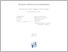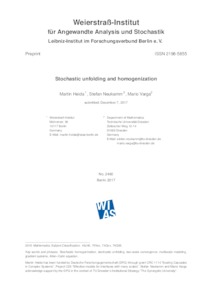Repository: Freie Universität Berlin, Math Department

# Stochastic unfolding and homogenization

Heida, M. and Neukamm, S. and Varga, M. (2017) Stochastic unfolding and homogenization. SFB 1114 Preprint at WIAS 12/2017 . pp. 1-45. (Unpublished)Preview

611kB

## Abstract

The notion of periodic two-scale convergence and the method of periodic un- folding are prominent and useful tools in multiscale modeling and analysis of PDEs with rapidly oscillating periodic coe cients. In this paper we are interested in the theory of stochastic homogenization for continuum mechanical models in form of PDEs with random coe cients, describing random heterogeneous materials. The notion of periodic two-scale convergence has been extended in di erent ways to the stochastic case. In this work we introduce a stochastic unfolding method that fea- tures many similarities to periodic unfolding. In particular it allows to characterize the notion of stochastic two-scale convergence in the mean by mere convergence in an extended space. We illustrate the method on the (classical) example of stochastic homogenization of convex integral functionals, and prove a stochastic homogeniza- tion result for an non-convex evolution equation of Allen-Cahn type. Moreover, we discuss the relation of stochastic unfolding to previously introduced notions of (quenched and mean) stochastic two-scale convergence. The method descibed in the present paper extends to the continuum setting the notion of discrete stochastic unfolding, as recently introduced by the second and third author in the context of discrete-to-continuum transition.

Item Type: Article Mathematical and Computer Sciences > Mathematics > Applied Mathematics 2210 Silvia Hoemke 15 Feb 2018 15:18 15 Feb 2018 15:37

Repository Staff Only: item control page